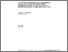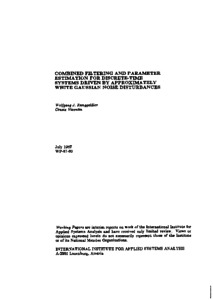# Combined Filtering and Parameter Estimation for Discrete-Time Systems Driven by Approximately White Gaussian Noise Disturbances

Runggaldier, W.J. & Visentin, C. (1987). Combined Filtering and Parameter Estimation for Discrete-Time Systems Driven by Approximately White Gaussian Noise Disturbances. IIASA Working Paper. IIASA, Laxenburg, Austria: WP-87-060Preview Text WP-87-060.pdf Download (411kB) | Preview

## Abstract

In the problem of combined filtering and parameter estimation one considers a stochastic dynamical system whose state x_t is only partially observed through an observation process y_t. The stochastic model for the process pair (x_t, y_t) depends furthermore on an unknown parameter theta. Given an observation history of the process y_t, the problem then consists in estimating recursively both the current state x_t of the system (filtering) as well as the value theta of the parameter (Bayesian parameter estimation).

The problem is a rather difficult one: Even if, conditionally on a given value of theta, the process pair (x_t, y_t) satisfies a linear-Gaussian model so that the filtering problem for x_t can be solved via the familiar Kalman-Bucy filter; when theta is unknown, the problem becomes a difficult nonlinear filtering problem.

The present paper, partly based on previous joint work of one of the authors, makes a contribution towards the solution of this problem in the case of discrete time and of a (conditionally on theta) linear model for x_t, y_t. The solution that is obtained is shown to be robust with respect to small variations in the a priori distributions in the model, in particular those of the disturbances.

Item Type: Monograph (IIASA Working Paper) Adaption and Optimization (ADO) IIASA Import 15 Jan 2016 01:58 27 Aug 2021 17:13 https://pure.iiasa.ac.at/2992View Item